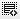Discounted Cash Flow0
Why is D&A not added to the EBITDA and instead subtracted?

(since in the analysis we had added it , Operating Income + D&A = EBITDA)0

The option "D&A should be subtracted from EBITDA" basically indicates what is done in the video were we start with EBIT (hence D&A is already subtracted from EBITDA) to show the unlevered net income.

Fundamentally this is how you calculate Unlevered FCF (UFCF)
1. Start with Earnings before interest and taxes (EBIT) x (1-Tax Rate)
2. Add back any dep/amortization (non cash expenses)
3. Subtract any changes in NWC (CA-CL)
4. Subtract any capex
5. Arrive at FCF

Now you will notice that the tax amount is applicable to EBIT and not EBITDA. Thats why first we start with EBIT (without the DA), then apply the tax rate to the EBIT, then add back D&A to reflect UFCF.

Unlike what you said in your answer, you cant just start with EBITDA (just because you are adding D&A back). If you start with EBITDA then the effective tax amount (lets say 30% of EBITDA) is different from the correct tax amount (30% of EBIT). So we start with EBIT, then apply the tax rate on this amount, then add back D&A.

Is this explanation helpful?

Click on thisicon to add code snippet.